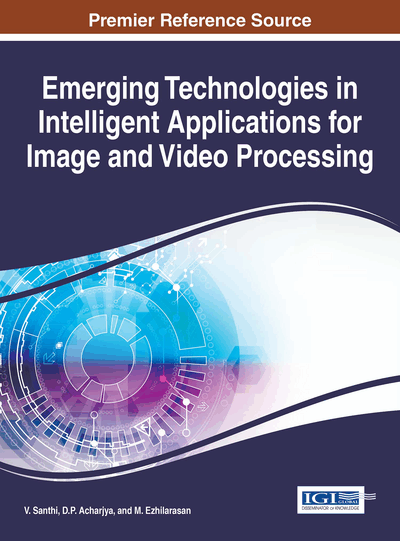# Indexing of Image Features Using Quadtree

N. Puviarasan (Annamalai University, India) and R. Bhavani (Annamalai University, India)
DOI: 10.4018/978-1-4666-9685-3.ch008

## Abstract

In Content based image retrieval (CBIR) applications, the idea of indexing is mapping the extracted descriptors from images into a high-dimensional space. In this paper, visual features like color, texture and shape are considered. The color features are extracted using color coherence vector (CCV), texture features are obtained from Segmentation based Fractal Texture Analysis (SFTA). The shape features of an image are extracted using the Fourier Descriptors (FD) which is the contour based feature extraction method. All features of an image are then combined. After combining the color, texture and shape features using appropriate weights, the quadtree is used for indexing the images. It is experimentally found that the proposed indexing method using quadtree gives better performance than the other existing indexing methods.
Chapter Preview
Top

Many approaches are devised to index the large databases. In spatial access indexing method, an image is represented using image features and different distances are used to find the similarity between the query image and database images. KD-Tree by Bentley et.al (1975) and R-Tree by Guttman et.al (1984) are the examples of spatial access method. An R-tree is a height-balanced tree similar to B-tree with index records are in its leaf nodes containing pointers to data objects. The structure of R tree which is designed so that a spatial search requires visiting only a small number of nodes, the index is completely dynamic. Then, R* Tree discussed by Beckmann et.al (1990) gives better performance than the R-Tree. R*-tree incorporates a combined optimization of area, margin and overlap of each enclosing rectangle in the directory. Rb-Tree was introduced by Sellis et.al (1987) used a method called ‘forced reinstart’.

The nearest neighbour search algorithms based on kd-trees, have been applied to large scale indexing and searching was found by Arya et.al (1998) and Lowe et.al (2004). Octavian et.al (2003) proposed Bkd- tree which is the extension of kd-tree is proposed to make static structure of tree to be dynamic. Complete survey of multi-dimensional indexing are found by Gaede et.al (1998) and Arge et.al (2002). The authors Berg et.al (2008) and Shakhnarovich et.al (2006) discussed that the nearest neighbour (NN) search is a fundamental problem in the research communities of computational geometry and machine learning. The k-D-B Tree is proposed by Robinson (1981). It is a height-balanced tree similar to the B+tree and its tree structure is constructed by dividing the search space into sub regions with coordinate planes recursively.

The TV-Tree using telescope vectors is devised by Lin et.al (1994). It organises the data in hierarchical structure. Berchtold et.al (1996) introduced X-Tree for indexing high-dimensional data. It uses the split algorithm minimizing overlap and additionally utilizes the concept of supernodes. It performs better than TV-Tree and R* Tree. RP-KD Tree is proposed by Pengcheng et.al (2011) in which multiple KD trees are used to present the data points in CBIR system into a lower-dimensional space. Space partitioning structures like quadtree by Samet (1984) and LSD Tree by Andreas et.al (1989) are used for high-dimensional feature space. A variant optimized k-d tree called VAM k-d tree is presented by White et.al (1997). A new dynamic index structure called GC-tree is proposed by Guang-Ho Cha et.al (2002). It is based on subspace partitioning method optimized for high-dimensional image dataset.

## Complete Chapter List

Search this Book:
Reset